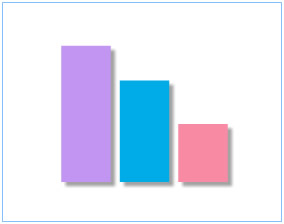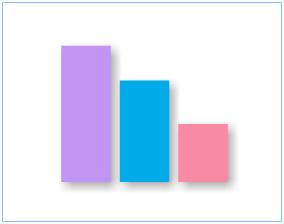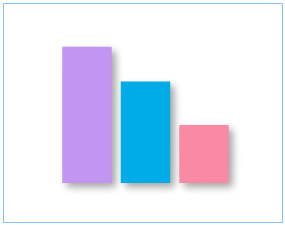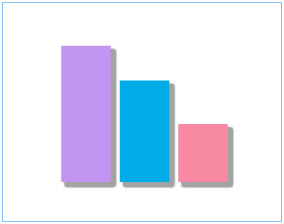You are viewing documentation for an older version. For current documentation - click here.

The Shadow Style Type helps you apply shadow effect to any of the chart objects.Note: In JavaScript charts, customized shadow style type is not available.

It extends the following configurable properties:

 Properties Description distance The offset distance for the shadow (in pixels). The default value is 4. angle The angle of the shadow. Valid values are 0 to 360˚. The default value is 45. color The color of the shadow in hex code (without #). The default value is CCCCCC. alpha The alpha transparency value for the shadow color. Valid values are 0 to 100. For example, 25 sets a transparency value of 25%. blurX The amount of horizontal blur. Valid values are 0 to 255. The default value is 4. Values that are a power of 2 (such as 2, 4, 8, 16 and 32) are optimized to render more quickly than other values. blurY The amount of vertical blur. Valid values are 0 to 255. The default value is 4. Values that are a power of 2 (such as 2, 4, 8, 16 and 32) are optimized to render more quickly than other values. strength The strength of the imprint or spread. The higher the value, the more color is imprinted and the stronger the contrast between the shadow and the background. Valid values are from 0 to 255. The default is 1. quality The number of times to apply the shadow effect. Valid values are 0 to 15. The default value is 1, which is equivalent to low quality. A value of 2 is medium quality, and a value of 3 is high quality. Shadow with lower values are rendered quicker.

The following code helps set the shadow distance and angle:

`<style name='MyFirstShadow' type='Shadow' distance='6' angle='45'/>`
`"definition":[{ "name":"MyFirstShadow", "type":"Shadow", "distance":"6", "angle":"45" }]`
 Property Format Example distance distance='value in pixels' distance='6' angle angle='value between 0 to 360' angle='45'

When applied on the columns of a 2D Column Chart, as under:

`<apply toObject='DataPlot' styles='MyFirstShadow' />`
` "application":[{ "toobject":"DataPlot", "styles":"MyFirstShadow" }]`

It gives the following output:You can set any distance (in pixels) and any angle between 0-360.

You can configure shadow color and alpha as under:

`<style name='MyFirstShadow' type='Shadow' color='D72060' alpha='30' distance='6' angle='45' />`
`"definition":[{ "name":"MyFirstShadow", "type":"Shadow", "color":"D72060", "alpha":"30", "distance":"6", "angle":"45" }]`
 Property Format Example color color='Hex value (without #)' color='D72060' alpha alpha='value (between 0 to 100)' alpha='30'

The above code changes shadow color to pink (D72060) with a transparency of 30% as under:You can configure the blur amount of shadow as under:

`<style name='MyFirstShadow' type='Shadow' distance='6' angle='45' blurX='14' blurY='14'/>`
`"definition":[{ "name":"MyFirstShadow", "type":"Shadow", "distance":"6", "angle":"45", "blurx":"14", "blury":"14" }]`
 Property Format Example blurX blurX='value' blurX='14' blurY blurY='value' blurY='14'(Original shadow – without any blur) (With blurX and blurY as 14)
Controlling strength and quality

The shadow's strength and quality can be controlled as under:

`<style name='MyFirstShadow' type='Shadow' distance='6' angle='45' quality='10'/>`
`"definition":[{ "name":"MyFirstShadow", "type":"Shadow", "distance":"6", "angle":"45", "quality":"10" }]`
 Property Format Example quality quality='value' quality='10' strength strength='value' strength='3'(With default quality) (With quality set as 10)

You can also set the strength of the shadow as under:

`<style name='MyFirstShadow' type='Shadow' distance='6' angle='45' strength='3'/>`
`"definition":[{ "name":"MyFirstShadow", "type":"Shadow", "distance":"6", "angle":"45", "strength":"3" }]`

This yields the following:(With default strength) (With strength set as 3)

Using a combination of the above discussed attributes, you can create shadow effects the way you like it!

Let's now shift focus to Glow Style Type.